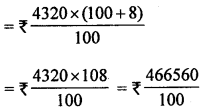## ML Aggarwal Class 8 Solutions for ICSE Maths Chapter 7 Percentage Ex 7.4

Question 1.
Find the buying price of each of the following when 5% S.T. is added on the purchase of
(i) a towel of ₹50
(ii) 5 kg of flour at ₹15 per kg.
Solution:
(i) S.T. = 5%
Cost of towel = ₹50
Totals. S.T. = ₹ $$\frac{50 \times 5}{100}$$ = ₹ 2.50
∴ Buying price = ₹50 + ₹2.50 = ₹52.50

(ii) Cost price of 5 kg of flour at the rate for ₹15 perkg = ₹15 × 5 = ₹75
Rate of S.T. = 5%
∴ Total tax = $$\frac{75 \times 5}{100}$$ = ₹ $$\frac{375}{100}$$ = ₹3.75
Total price of the flour = ₹75 + ₹3.75 = ₹8.75

Question 2.
If 8% VAT is included in the prices, find the original price of
(i) a TV bought for ₹ 13500
(ii) a shampoo bottle bought for ₹ 180.
Solution:
(i) Total price of a TV including VAT = ₹13500
Rate of VAT = 8%
Original price of TV = ₹ $$\frac{13500 \times 100}{100+8}$$ = ₹ $$\frac{13500 \times 100}{108}$$ = ₹12500
(ii) Total cost of shampoo bottle including VAT = ₹180
Rate of VAT = 8%
∴ Original price of shampoo = ₹ $$\frac{180 \times 100}{100+8}$$
= ₹ $$\frac{180 \times 100}{108}$$ = ₹ $$\frac{500}{3}$$
= ₹ 166.67

Question 3.
Utkarsh bought an AC for ₹34992 including a VAT of 8%. Find the price of AC before VAT was added.
Solution:
Cost of AC including VAT = ₹34992
Rate of VAT charged = 8%
∴ Original price of AC = ₹ $$\frac{34992 \times 100}{100+8}$$ = ₹ $$\frac{34992 \times 100}{108}$$ = ₹ 32400

Question 4.
Gaurav bought a shirt for ₹ 1296 including VAT. If the original price of the shirt is ₹ 1 200, find the rate of VAT.
Solution:
Cost of shirt including VAT = ₹ 1296
Actual price of shirt = ₹120
∴ Amount of VAT = ₹ 1296 – 1200 = ₹ 96
Rate of VAT = $$\frac{\mathrm{VAT} \times 100}{\mathrm{C.P.}}$$
= $$\frac{96 \times 100}{1200}$$ = 8%

Question 5.
Anjana buys a purse for ₹523.80 including 8% VAT. Find the new selling price of the purse if VAT increases to 10%.
Solution:
Total cost price of purse including VAT = ₹ 523.80
Rate of VAT = 8%
∴ Actual cost of the purse = ₹ $$\frac{523.80 \times 100}{100+8}$$
= ₹ $$\frac{523.80 \times 100}{108}$$ = ₹ 485
New rate of VAT = 10%
Amount of VAT = ₹ 485 × $$\frac{10}{100}$$ = ₹ $$\frac{4850}{100}$$ = ₹48.50
∴ Total cost of the purse = ₹485 + ₹48.50 = ₹535.50

Question 6.
A wall hanging is marked for ₹4800. The shopkeeper offers 10% discount on it. If VAT is received 8% from the customer, find the amount paid by the customer to purchase the wall hanging.
Solution:
Marked price of wall hanging = ₹ 4800
Discount offered = 10%
∴ Net sale price = ₹ $$\frac{4800 \times(100-10)}{100}$$
= ₹$$\frac{4800 \times 90}{100}$$ = ₹ 4320
Rate of VAT charged = 8%
Sale price including VAT= ₹ 4665.60

Question 7.
Amit goes to a shop to buy a washing machine. The marked price of the washing machine is ₹ 10900 excluding9% VAT. Amit bargains with the shopkeeper and convinces him for ₹10900 including VAT as the final cost of the washing machine. Find the amount reduced by the shopkeeper.
Solution:
Marked price of washing machine = ₹ 10900
Rate of VAT = 9%
Let the reduced price of machine = ₹ x
VAT at the rate of 9% = ₹ x × $$\frac{9}{100}$$ = ₹ $$\frac{9x}{100}$$
Amount paid = ₹ x + $$\frac{9x}{100}$$ = $$\frac{109}{100}$$ x
∴ $$\frac{109}{100}$$x = ₹ 10900 ⇒ x = $$\frac{10900 \times 100}{109}$$
∴ x = 10000
∴ Amount reduced by the shopkeeper = ₹10900 – 10000 = ₹900